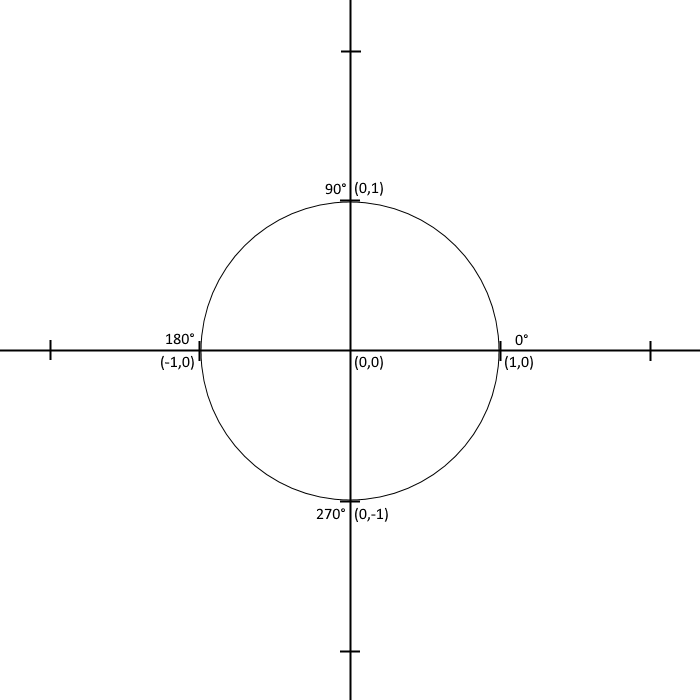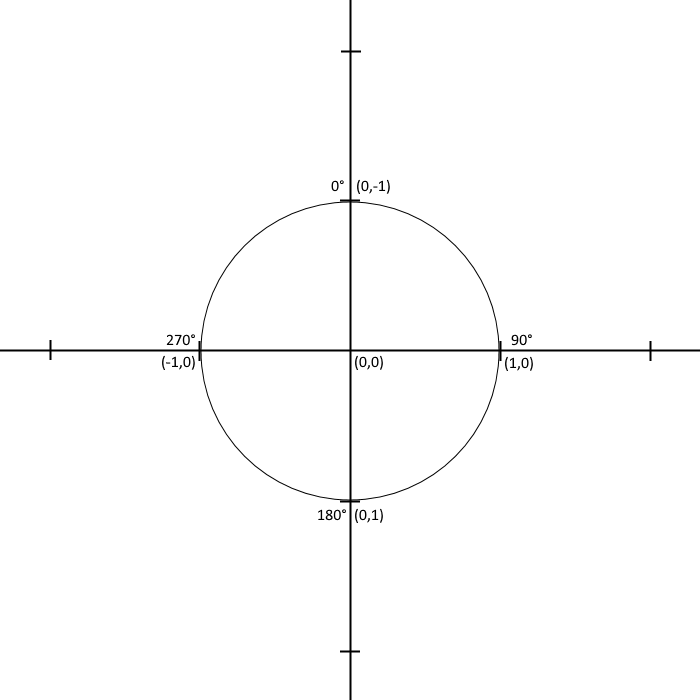November 10th, 2019

Last friday, a friend of mine came over in the evening for a coding party. We shared with each other what we were working on, bounced ideas and problems off each other, listened to some Two Steps from Hell, and of course wrote plenty of code.

I shared with him the big issue I've been dealing with in the game I am building: I created a turret that is supposed to track and shoot at the player's spaceship, but it's aim is off by 90 degrees. While discussing what could be going on, my friend brought up the unit circle we all learned about in trigonometry class.

To refresh your memory, below is an example of a unit circle.This circle is useful for visualizing the relationship between angles and coordinates on an XY plane. For example, the angle 180 degrees points to the left, the negative X direction. If you calculate the cosine of 180 degrees, you will get a result of -1, and the sine of 180 degrees will result in 0. This matches what we see on the unit circle. So, cosine gives the X coordinate and sine gives the Y.

This makes it easy to calculate a vector given an angle. Unless.... Unless you are working with a coordinate system which is different than the unit circle above. My friend and I figured out I was working with a coordinate system that is different than the standard one in several ways.

• 0 degrees is up instead of right.
• Y increases down instead of up.
• The angle increases clockwise instead of counter-clockwise.

A unit circle for this system looks like this:How do we calculate XY coordinates from an angle in this system? We need to figure out the transform between the standard system and Phaser's system. Take the right direction for example; it is now 90 degrees instead of the standard 0. We know from the standard unit circle that cos and sin of 90 degrees make 0 and 1, respectively, the opposite of what we need for Phaser's coordinate system. So, we swap them; set X equal to sine instead of cosine, and set Y equal to cosine instead of sine. Next we try another angle, 180 degrees. Sine/cosine gives (-1, 0). We apply the current transform and get (0, -1). The sign of Y is still wrong, so we multiply Y by -1. This gives us (0, 1), what we want. Continuing around the circle, 270 degrees is (0, -1), transformed is (-1, 0). o degrees is (1, 0), transformed is (0, -1). The transform works!

I applied this transform to my calculations in my game and everything worked! Well, not everything, but my enemy turrets were suddenly very accurate.

The lesson I learned from this: know your coordinate system and how to transform the results of sine/cosine to work within it.